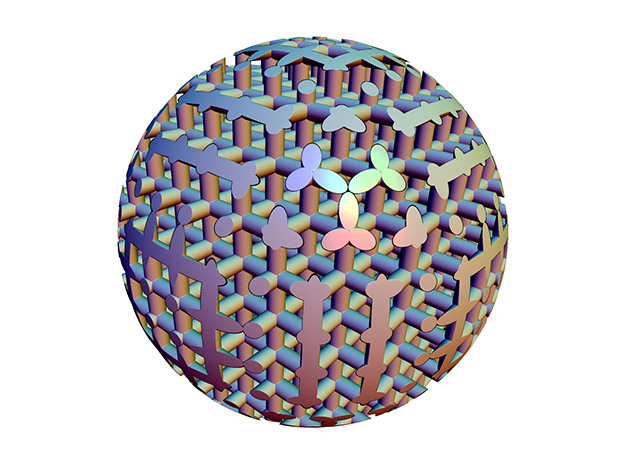For this week we’ll start into the powerful world of symmetry and reflections. ShapeJS allows arbitrary transformations of objects and as we’ll see in this post this has great power and some sharp edges to avoid. Guest writing this week is Vladimir Bulatov, he’s the math whiz behind the curtains of ShapeJS.

PeriodicWrap is a ShapeJS transformation which is most useful for creating repeated shapes and patterns. However if used incorrectly it can produce unexpected results. The purpose of this tutorial is to provide basic info and examples with correct use of PeriodicWrap, highlight potential problems and suggest possible solutions.

PeriodicWrap generates a fill of the three dimensional space (tiling) with copies of a single tile – the fundamental domain. PeriodicWrap is defined by linearly independent basis vectors and an origin. All parameters have type Vector3d. Basis vectors define the shape and orientation of the fundamental domain and the origin defines the place of the fundamental domain in space. There are 3 variants of PeriodicWrap one-, two- and three-periodic. This week we’ll examine the one periodic version, and in a later post we’ll cover the more complex versions.

One-periodic wrap
We start with the simplest variant – one-periodic. It is defined by one basis vector and origin. The origin has default value (0,0,0) which means the PeriodicWrap is located at the origin of the coordinate axes.

```var a = 5*MM;
var pw = new PeriodicWrap(new Vector3d(a,0,0));
```

This creates a one dimensional PeriodicWrap with basis vector (a,0,0).The one-periodic tiling can be imagined as cutting a space into set of identical slices. The fundamental domain (yellow) is a single slice. Slices are orthogonal to the basis vector (in this case (a,0,0). The thickness of the slice equals the length of the basis vector (5mm in this case) and the left side of the slice passes through the origin of the PeriodicWrap (in this case default value (0,0,0)). Let’s place a simple shape inside of the fundamental domain.

```  var sphere = new Sphere(a/2,a/2,0, a/3); // sphere radius a/3 centered at (a/2,a/2, 0)
```And apply PeriodicWrap to the shape.

```  sphere.setTransform(pw); // tiled sphere
```

Here is the diagram of the result:Shapes are replicated in both directions indefinitely. Below is the screenshot of the ShapeJS rendering:Let’s place a different shape in a different spot

```  var redSphere = new Sphere(0,a/3,0,a/2); // sphere radius a/2 centered at (0,a/3,0)
```PeriodicWrap works by filling the whole space by identical copies of single tile – the fundamental domain.The interior of the fundamental domain is replicated. Parts of the shape outside of fundamental domain are cropped. It means, that the half of sphere sitting outside of fundamental domain abruptly disappears.The rendering looks even worse, the cropping induces severe rendering artifactsThere is a simple solution to the problem. We can shift the origin of the tiling to make a shape enclosed by the fundamental domain

```  pw.setOrigin(new Vector3d(-a/2,0,0));
```And here is the tilingThe rendering now looks correct as wellBut it is not always possible to select a good location for the tilings origin. In example below we have a union of two shapes.The union doesn’t fit inside of fundamental domain. Therefore the shape always will be partially cropped:The solution to the problem is to build a shape inside of the fundamental domain in a way which will compensate for unavoidable cropping: the opposite sides of fundamental domain have to pair seamlessly to each other. In this case we can place copy of another red sphere translated by the tiling basis vector (a,0,0)Here are the drawing and rendering of the resulting tiling:Complete ShapeJS script of the example with two shapes:

```function main(args) {
var w = 30*MM; // scene size
var a = 5*MM; // period of wrap
var s1 = new Sphere(a/2, a/2, 0, a/3);  // shape 1
var s2 = new Sphere(0, 0.7*a, 0, a/2);  // shape 2
var s2t = new Sphere(a, 0.7*a, 0, a/2); // translated shape 2
var pw = new PeriodicWrap(new Vector3d(a,0,0));
var shape = new Union(s1, s2);
shape.setTransform(pw);
return new Scene(shape,new Bounds(-w,w,-w,w,-w,w));
}
```

After a PeriodicWrap transform is applied to a shape inside of the fundamental domain we normally obtain an unbounded shape. That shape intersects the scene bounds and as result has an open interior, which results in manifold problems in the generated mesh as is shown below with red.In order to obtain a finite shape of specific size, we may intersect unbounded shape with some shape of specific size, for example a box:

```shape = new Intersection(shape, new Box(55*MM, 20*MM, 20*MM));
```PeriodWraps are a powerful method for creating many copies of your geometry. It’s also a much more efficient way to create lots of shapes instead of copying the datasources. In our next posting on symmetry and reflections we’ll explore the two and three periodic wrappings. These allow you to create some interesting patterns, similar to what you might find in nature. As a taste here are some two and three periodic wrappings: